menu 3 Newton's Law of GravitationErasmus+: Key action 2:Strategic Partnerships in School Education# Practical exercise 5: ESCAPE VELOCITY

## Aim:

To better understand the concept of escape velocity.

## Instructions for the teacher:

The information below needs to be understood very well by the students.

## Instructions for the student: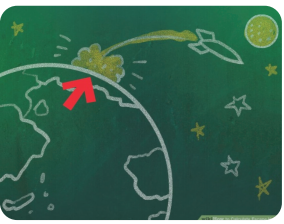In order to understand how the formula for escape velocity is derived, follow carefully the listed steps:

1. Define escape velocity: it is the velocity an object must have to overcome the gravitational pool of the planet it is on in order to “escape” into space. The larger planets have larger masses and require higher escape velocities that the smaller planets.

2. The conservation of energy law states, that the total energy of an isolated system does not change. If we consider the system Earth - rocket as isolated, in the conservation of energy law the sum of the initial potential (U) and kinetic (K) energies (indexed 1) has to be equal to the sum of the resultant potential and kinetic energies (indexed 2) :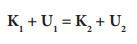3. Define potential and kinetic energy. Kinetic energy is energy of motion К = (½) m v2, where m is the mass of the rocket and v is its velocity. The potential energy depends on the placement of and object in respect to the other objects in the system. In physics, the potential energy of a body that is infinitely far away from Earth usually is said to be zero. Since the gravitational force is a force of attraction, the potential energy of the rocket will be negative and decreases when the rocket gets closer to Earth. The potential energy of the system Earth - rocket can be written as: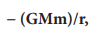where G is the gravitational constant, M is the mass of the Earth, m – mass of the rocket, and r – is the distance between the centres of the two masses.

4. We use these energies in the conservation of energy law. Assume the rocket’s velocity is the minimal velocity, needed to overcome the Earth’s gravitational pull. This velocity will constantly decrease until in some moment it will become zero (at infinitely distant point, relative to Earth). So we take К2 = 0. At this time the rocket will not feel the Earth’s gravitation and will never “fall” back to Earth. So U2 = 0 too. Then: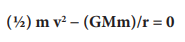5. We solve the above equation for v: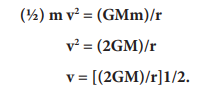This is the escape velocity in this case, i.e. the minimal velocity that the rocket needs to overcome the gravitational pull of the Earth and fly out to space.